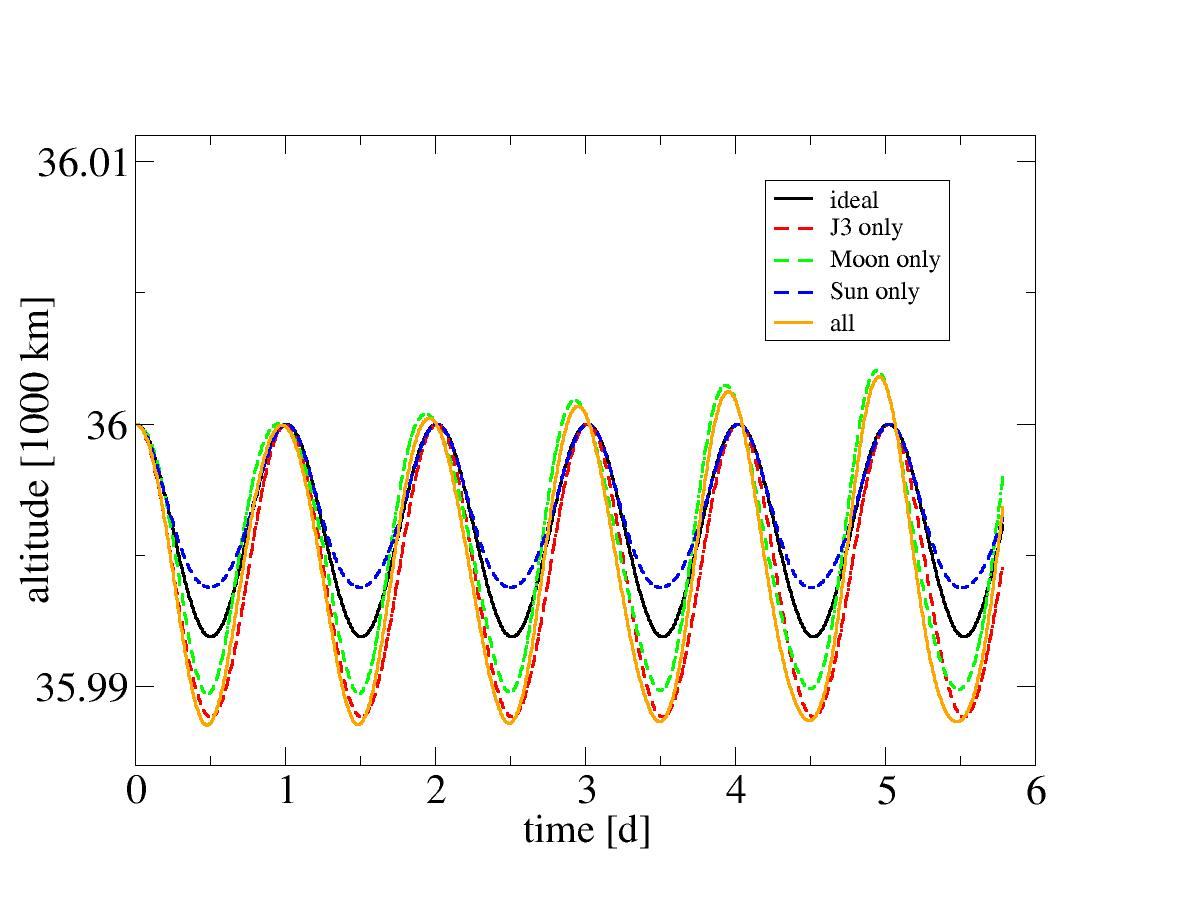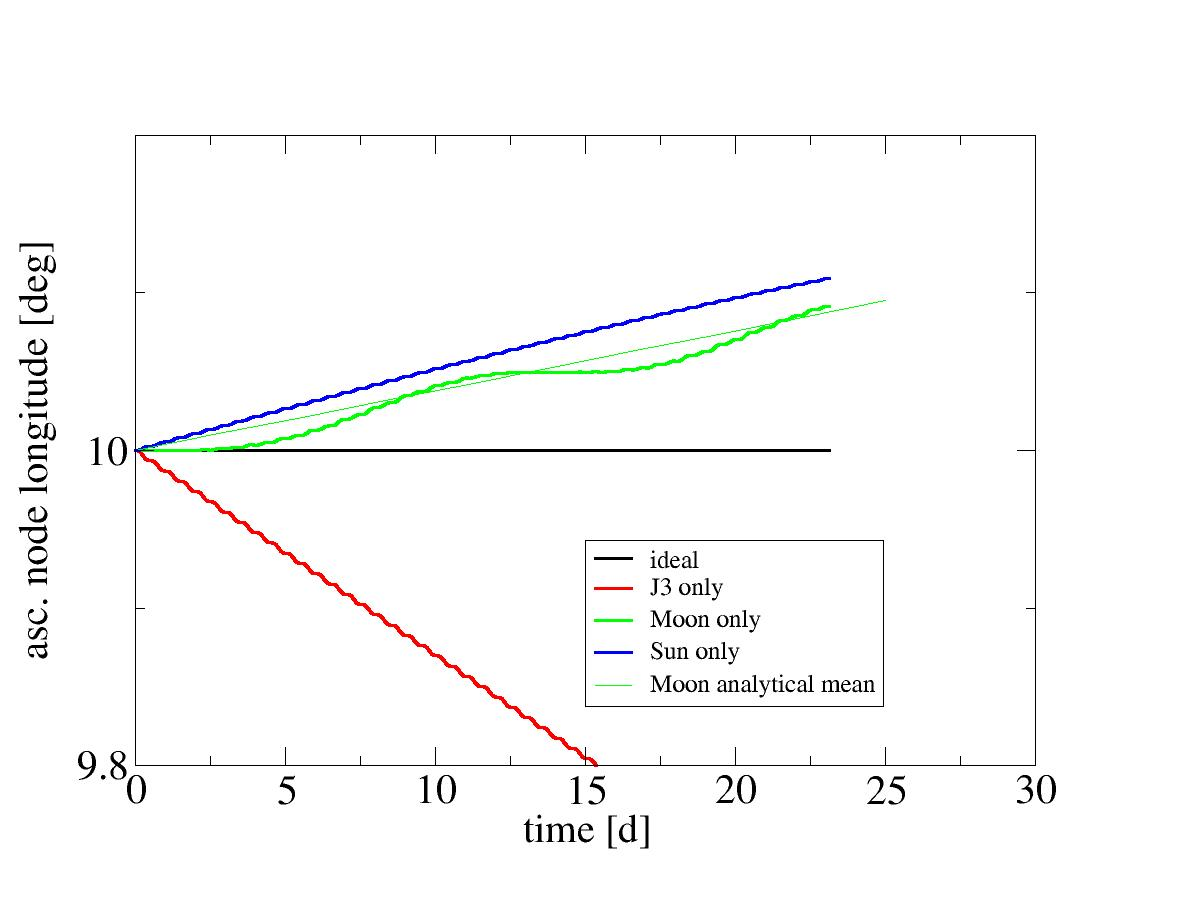# Multibody perturbations

Let us now get back into Earth orbit and ask how the gravity of Moon (and Sun) affects such orbits. Of course the gravity of the Moon pulls at a spacecraft in Earth orbit - but it also pulls at Earth with the same acceleration, so if Earth is used as the coordinate reference, to first approximation there is no effect. Only to second approximation the gravity field at the position of the spacecraft is slightly different from the gravity field at the center of Earth, and this is where the perturbation comes from.

The perturbing field strength hence varies periodically - whenever the spacecraft is closer to the Moon than Earth, there is a net pull towards the Moon, and the situation is reversed when the spacecraft is on the portion of the orbit behind Earth. Unlike the other perturbations we've looked at so far (the J2 and the J3 terms mostly), the perturbation is not radially symmetric around Earth's rotation axis - the orientation relative to the direction to the Moon matters.

For this reason, there is a second pronounced effect to be expected - a longer periodicity having to do with the the movement of the Moon around Earth (or of Earth around the Sun) which slowly rotates the direction to the perturbing body in Earth-relative coordinates. For the same reason, we can also expect that at a given instance of time, the perturbation observed is rather different dependent on e.g. the longitude of the ascending node. Generally, in this situation the mean perturbation becomes only a meaningful concept over large averaging times, even if the mean perturbation in an element is zero, the actual deviation from an unperturbed orbit at a given time may be comparatively large.

## Effects on position

Since the magnitude of the perturbation grows with distance to Earth, a geosynchronous orbit is affected much more by 3rd body perturbations than a low orbit. This is in fact the opposite trend to the J2 and J3 perturbations which are stronger in low orbits and gradually die out with increasing radius.

For this reason, we'll set up a fairly high orbit to study perturbations. Add the following setup to your config file

 config units SI gravity_model spherical earth_model spherical plot2d_resolution 40000 timestep 0.01 max_time 500000.0 position lat 0.0 lon 10.0 alt 36000000 heading 80.0 vup 0.0 vtot 3067

and run
 plot2d file alt_XX.dat x time y altitude

for the output, using first just spherical gravity, then J3 gravity, and then spherical gravity with the Moon added and with the Sun added - the latter can be done via
 celestial_body name Sun ephemeris ephemerides/ephemeris_sun.dat GM 1.327124400e20

if you don't know the GM value offhand. The result, plotted into the same graph, should give you something like this:Perturbations of position due to Sun and Moon.

For this orbit, the effects are roughly of the same magnitude, i.e. O(10) km over a few days. For the time period considered, the drift of the periapsis location due to the movement of the Moon is clearly observable, though the time run is not long enough to see a full revolution of the Moon around Earth (much less to see apparent movement of the Sun).

## Drift of the ascending node

One of the elements that changes in a non-periodic manner with the perturbation is the longitude of the ascending node. The expected mean drift rate due to the Moon is 0.0038 degrees per day times the inclination cosine for an orbit at geosynchronous altitude. We can plot the drift due to various effects as compared with an analytical orbit and also compare with the analytical value.Perturbations of position due to Sun and Moon.

It becomes apparent quickly that the actual drift rate is highly variable dependent on whether the Moon is over the node or not. At the timescale of a month plotted, the Sun doesn't show any such periodicity yet (and would only be expected to when looking at a six month period) and in fact for the orbit chosen is nearly at its maximum (you can easily verify that if you move the starting longiude of the orbit by 90 degrees, the Sun-induced drift will be much slower and in fact be close to the minimum). For that reason, a comparison with the analytically expected average rate of 0.00154 degrees per day is difficult.

Based on the analytical expression, the expected drift rate for lower orbits is to be divided by the number of revolutions per day, i.e. for a LEO effects can be expected to be down by a factor ~15 or so, making 3rd body perturbations much subleading to the J2 and J3 effects there.

Continue with Rendezvous basics.

Back to main index     Back to science     Back to LEO targeting

Created by Thorsten Renk 2018 - see the disclaimer, privacy statement and contact information.